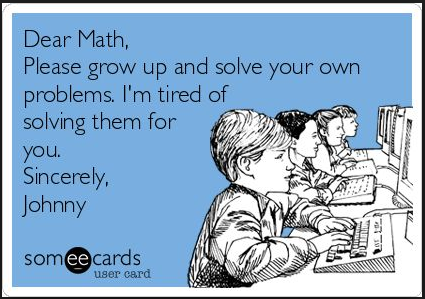Solving Systems of Equations
4 years ago
caityarmstrong
Save
Edit
Host a game
Live GameLive
Homework
Solo Practice
Practice20 QuestionsShow answers
• Question 1
300 seconds
Q. Solve for x and y.
y = 2/3x - 2
y = -x + 3
(0,3)
(0,-3)
(3,0)
(-3,0)
• Question 2
300 seconds
Q. Solve for x and y
3x + 2y = 16
7x + y = 19
(-2,5)
(-2,-5)
(2,-5)
(2,5)
• Question 3
300 seconds
Q.

2x - y = 0

4x - y = 0

(1,2)

(0,0)

No Solutions

Infinite Solutions

• Question 4
300 seconds
Q. In a pasture are horses and chickens if there are a total of 28 animals and 80 legs how many chickens and horses?
10 horses, 18 chickens
16 horses, 12 chickens
12 horses, 16 chickens
18 horses, 10 chickens
• Question 5
300 secondsQ. What is the solution?
A
B
C
D
• Question 6
300 seconds
Q. Erin is 3 years younger than twice Alex's age. Their ages combined are 33 years. How old are Alex and Erin. If x=Erin's age and y=Alex's age, choose the system that matches the situation.
x + y = 33
y = 2x - 3
x + y = 33
x = 2y - 3
x + y = 33
x = 3 - 2y
x + y = 3
x = 33 - 2y
• Question 7
300 seconds
Q. The equations of two lines are:
2x-y=4 and y=-2x+8.
What is the value of x in the solution for this system?
x=8
x=3
x=11
x=5
• Question 8
300 seconds
Q. Christian had brochures printed for a new business venture. Christian originally ordered 4 boxes of black-and-white brochures and 3 boxes of color brochures, which cost a total of \$134. After those ran out, Christian spent \$120 on 3 boxes of black-and-white brochures and 3 boxes of color brochures. Which system represents this situation?
x+y=134
x+y=120
3x+3y=134
4x+3y=120
4x+3y=134
3x+3y=120
7xy=134
6xy=120
• Question 9
300 seconds
Q. Two brothers went shopping at a back-to-school sale where all shirts and shorts were the same price. The younger brother spent \$175 on 7 new shirts and 7 pairs of shorts. The older brother purchased 6 new shirts and 7 pairs of shorts and paid a total of \$165. How much did one shirt cost?
\$5
\$10
\$15
\$20
• Question 10
300 seconds
Q. David is running a concession stand at a soccer game. He sells nachos and sodas. Nachos cost \$1.50 each and sodas cost \$0.50 each. At the end of the game, David made a total of \$78.50 and sold a total of 87 nachos and sodas combined. Which system of equations represents this situation?
1.5x+0.5y=78.5
x+y=87
1.5x+0.5y=78.5
1.5x+0.5y=87
x+y=78.5
1.5x+0.5y=87
x+y=78.5
x+y=87
• Question 11
120 seconds
Q. If a system of equations has no solution, what does the graph look like?
intersecting lines
parallel lines
skew lines
intersecting lines
• Question 12
300 seconds
Q. Does the system have one, none or infinite solutions?
8x + 4y = 12
y = -2x + 3
(0,3)
(3,0)
No solution(parallel lines)
Infinitely many solutions
• Question 13
300 seconds
Q. What is the value of the y-coordinate of the solution to the system of equations
x-2y=1
x+4y=7
1
-1
3
4
• Question 14
300 seconds
Q. Caitlin won a bag full of money! She has 49 bills in all. She counts \$1,430. There are \$20 dollar bills and \$50 dollar bills. How many of each bill does Caitlin have?
WRITE a system where T= # of \$20 and F = # of \$50.
T + F = 1430
20T + 50F = 49
T+ F = 49
10T + 5F = 1430
T + F = 49
20T + 50F  = 1430
T + F = 49
T + F = 1430
• Question 15
300 secondsQ. Write the inequalities represented in the picture.
y > -2 + x
y ≤ 2x + 2
y > 2 + x
y ≤ 2x + 2
y ≥ -2 + x
y ≤ 2x + 2
none of these are the system
• Question 16
300 secondsQ. Write the inequality represented in the picture.
x ≥ -1
x ≤ -1
x > -1
x < -1
• Question 17
300 secondsQ. Write the inequalities represented in the picture.
y ≤ 3x   and  y ≤ -2x + 4
y > 3x   and  y > -2x + 4
y < 3x   and  y > -2x + 4
y > 3x   and  y < -2x + 4
• Question 18
300 secondsQ. Write the inequalities represented in the picture.
y > -4/5x - 1 and y ≤ 2/3x + 3
y ≥ -4/5x - 1 and y ≤ 2/3x + 3
y < -4/5x - 1 and y < 2/3x + 3
y < -4/5x - 1 and y ≥ 2/3x + 3
• Question 19
60 secondsQ. What is the solution?
One Solution
No solution
Infinitely Many Solutions
• Question 20
60 secondsQ. What is the solution?